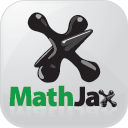# The Math View

The math view presents formulas as they would appear in a mathematics text. For example, the math view for the quadratic formula

(-B3 + SQRT(B3^2-4*A3*C3))/(2*A3)

employs the usual mathematical typesetting conventions for fractions, squares, and square roots.Math view of the quadratic formula

## Typesetting conventions

The math view uses the following conventions when typesetting formulas. Here, x, y, and z represent arbitrary expressions that are typeset as $x$, $y$, and $z$.

Math View Typesetting
Expression Typeset
Product: x*y $x\cdot y$
Simple fractions: x/y, (x)/y,
x/(y), (x)/(y)
$\frac{x}{y}$
Fraction in a numerator: x/y/z $\frac{x}{y\cdot z}$
Simple power: x^y ${x}^{y}$
Power of a power: x^y^z ${x}^{y\cdot z}$
Less than or equal to: x<=y $x\le y$
Not equal to: x<>y $x\ne y$
Greater than or equal to: x>=y $x\ge y$
Range union: x, y $x\cup y$
Range intersection: x y $x\cap y$
ABS(x) $|x|$
EXP(x) ${e}^{x}$
INT(x) $⌊x⌋$
LOG10(x) ${log}_{10}\left(x\right)$
PI() $\pi$
SQRT(x) $\sqrt{x}$

## Typesetting technologyMathJax is a display engine for mathematics. It takes input marked up in MathML, TeX, or ASCIImath, and it produces typeset output that displays well in any browser.

Formula Forge uses MathML as its markup language.